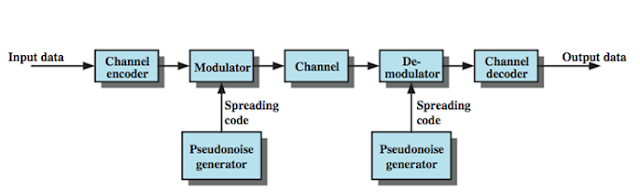## Pages

Overview :

Spread spectrum is a one types of techniques it can be used for transmitting radio or telecommunication signal. The increasing demand of wireless communication have faced problems because of limited spectrum capacity and multi-path propagation. These problems can be minimized by spread spectrum communication.

• Capability of multiple access
• Resistant to jamming
• It has the ability to resist multi path propagation
• Because of multi-path propagation. It provide  immunity to distortion
• Higher channel capacity
• It can not be intercepted by any unauthorized person

Figure shows the general block diagram of a spread spectrum digital communication system. so not let us talk about one by one block in spread spectrum model in detail.

To shown in figure the input is given to a channel encoder that produces the analog signal with narrow bandwidth at the center frequency.

The the signal is modulated by a spreading code or sequence. The spreading code is generated by pseudo noise or pseudo random number generator.

Modulation increases the bandwidth of the signal to be transmitted. At the receiver end the digit sequence is used to demodulate the spread signal. The signal is sent to the recover the data.Spread spectrum model
• Reduced cross talk interference
• Inherent security
• Hard to detect
• Longer operating distance
• Better voice quality
• Harder to jam
• Interception of signal will be difficult
• Privacy due to pseudo-random code
• Co-existence
Processing gain of spread spectrum :

The processing gain is defined as the factor by which the bandwidth of the message signal is increased.

Processing gain : N = Bss / B

Where,
B = Message signal bandwidth
Bss = Spread spectrum signal bandwidth

• Prevent signal jamming
• Cellular technology
• Cell phones
• Global positioning system
• Wireless local are network security
• Prevent Interference at specific frequency
• In obtaining the message signal
You may also check it out difference between DSSS and FHSS
Overview :

Spread spectrum is a one types of techniques it can be used for transmitting radio or telecommunication signal. The increasing demand of wireless communication have faced problems because of limited spectrum capacity and multi-path propagation. These problems can be minimized by spread spectrum communication.

• Capability of multiple access
• Resistant to jamming
• It has the ability to resist multi path propagation
• Because of multi-path propagation. It provide  immunity to distortion
• Higher channel capacity
• It can not be intercepted by any unauthorized person

Figure shows the general block diagram of a spread spectrum digital communication system. so not let us talk about one by one block in spread spectrum model in detail.

To shown in figure the input is given to a channel encoder that produces the analog signal with narrow bandwidth at the center frequency.

The the signal is modulated by a spreading code or sequence. The spreading code is generated by pseudo noise or pseudo random number generator.

Modulation increases the bandwidth of the signal to be transmitted. At the receiver end the digit sequence is used to demodulate the spread signal. The signal is sent to the recover the data.Spread spectrum model
• Reduced cross talk interference
• Inherent security
• Hard to detect
• Longer operating distance
• Better voice quality
• Harder to jam
• Interception of signal will be difficult
• Privacy due to pseudo-random code
• Co-existence
Processing gain of spread spectrum :

The processing gain is defined as the factor by which the bandwidth of the message signal is increased.

Processing gain : N = Bss / B

Where,
B = Message signal bandwidth
Bss = Spread spectrum signal bandwidth

• Prevent signal jamming
• Cellular technology
• Cell phones
• Global positioning system
• Wireless local are network security
• Prevent Interference at specific frequency
• In obtaining the message signal
You may also check it out difference between DSSS and FHSS